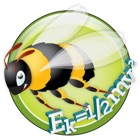EquationLab

• SEARCH TYPE

All iPhone applications categories

All iPhone games categoriesEquationLab

0 0

Screenshots

Description

Learn and master calculation skills in high school physics and maths, create perfectly laid out equation resources for documents and presentations, easily solve equation based problems for homework and class.

EquationLab can enrich a wide range of learning experiences where formula calculation is the focus, encouraging students to engage with this important area. It reinforces an effective calculation process and helps students become secure in their calculation skills. It's also a great productivity tool which saves valuable time when producing equation resources for documents and presentations.

Fast, intuitive drag and drop solving makes calculation easy:
• Select an equation, drag and drop to re-arrange, tap in values and get the answer.
• Solve equation calculations in a fraction of the time it takes with pen, paper and calculator.
• Enter values in equations first and then rearrange; this approach is favoured by many examination boards.

Interactive algebra tutor:
• Learning to rearrange equations can be a significant hurdle for many young people. Practising rearranging equations using EquationLab's powerful algebra tutor is a fun and engaging way for students to learn.

Perfectly laid out calculations - in seconds:
• Create perfect solutions and easily drop into documents or presentations.
• Email solutions to friends and colleagues.

Rich, colourful equation stories:
• Equation explanations and sample calculations show what each physics and maths equation is used for.

Prefixes, decimal places, significant figures and scientific notation:
• Interact with these important concepts.
• Immediately see the impact of changes in calculations and answers.

Virtual text book content covers all important equation concepts:
• Read about rearranging equations, prefixes, decimal places, significant figures and scientific notation.
• An in app data book lets you quickly look up all important constants.

Scientific calculator:
• Students will need a calculator in exams, so the app has one.

EquationLab helps parents, teachers and students:

Parents:
• Check your child's homework is correct.
• Give your child a resource to support conversation and learning at home.
• Help your child engage with maths and physics in a way which is fun and interactive.

Teachers:
• Enrich classwork and engage students with a ready made equation resource.
• Produce equation solutions for documents and presentations in a fraction of the time taken with other methods.

Students:
• Practise and interact with all important equation concepts.
• Model your written work on EquationLab's solutions, speed up learning and improve exam results.

** In App Purchase **

EquationLab can be downloaded free with 6 sample equations. An In App Purchase gives you the full version of the app which contains all 92 equations, equation stories and unlocks EquationLab's powerful interactive algebra tutor. Having completed the IAP, you will automatically get all future upgrades.

The full equation set includes:

• Maths: Fractions, percentages, circumferences and perimeters, areas, volumes, circle arc and sector, Pythagoras, line and gradient, trigonometry, sine and cosine rule, trigonometric area, roots of a quadratic equation.

• Physics: Mechanics, heat, waves, electricity, power and efficiency, optics, nuclear physics, gas laws and rotational motion.